Subject:
Geometry
Material Type:
Lesson Plan
Level:
Middle School
7
Provider:
Pearson
Tags:
7th Grade Mathematics, Measurement, Missing Angles
Language:
English
Media Formats:
Text/HTML

# Pre-Assessment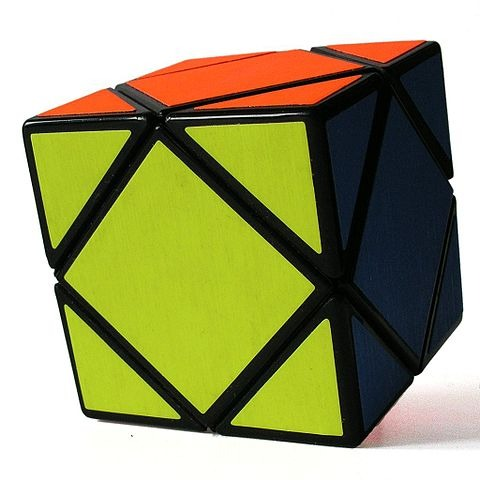## Overview

Students solve for missing angle measures by applying what they have learned about types of angles and the angle measures of polygons. Students do a pre-assessment at the end of the lesson.

# Key Concepts

There are many defining characteristics for angles, triangles, quadrilaterals, and polygons. Students have discovered these properties throughout this unit and have investigated why they are true. These characteristics and properties will be looked at more formally in high school geometry.

# Goals and Learning Objectives

• Solve for missing angle measures in polygons.

# Lesson Guide

After students view the opening figure, have them discuss the following questions:

• What do you know about the angles in this triangle?
• Can you use what you know to solve for x?

SWD: Help students with disabilities make connections between what they have learned in previous tasks about the interior angle sum of a triangle and writing algebraic equations.

# Mathematics

Students should notice the following:

• The sum of the measures of the angles of the triangle is 180°.
• The angle measures are 30°, 60°, and 90°.

$\begin{array}{ccccccc}x& +& 2x& +& 3x& =& 180°\\ & & & & 6x& =& 180°\\ & & & & x& =& 30°\end{array}$

Therefore:

2x = 60°

3x = 90°

# Angles in a Triangle

Look at this triangle.

• What do you know about the angles in this triangle?
• Can you use what you know to solve for x?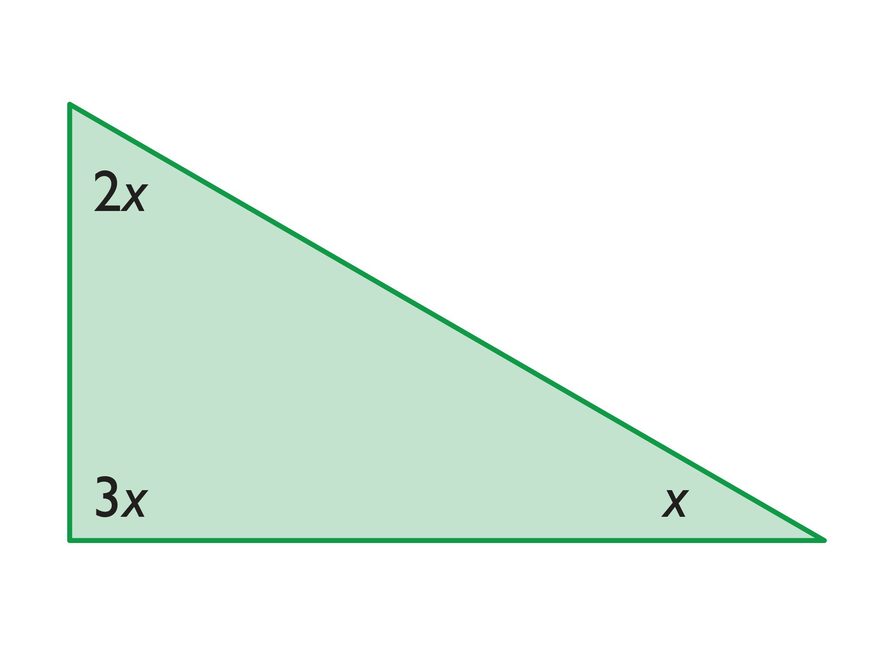# Lesson Guide

Discuss the Math Mission. Students will use their knowledge of geometric figures to determine missing angle measures in polygons.

## Opening

Use your knowledge of geometric figures to determine missing angle measures in polygons.

# Lesson Guide

Monitor students as they work, asking questions to guide them if they need assistance.

SWD: Students with disabilities may take longer to develop a solid understanding of newly introduced skills and concepts. Provide direct instruction and guided practice with the skills and concepts relating to interior angle sums. Assess their understanding of newly introduced concepts, plan a review, and reinforce skills as needed.

# Mathematical Practices

Mathematical Practice 1: Make sense of problems and persevere in solving them.

• Throughout the activities, students problem solve, make conjectures, and apply what they know.

# Interventions

Student has difficulty getting started.

• What do you know about the angles in a parallelogram?

• The measure of ∠a and ∠c is 30°. The measure of ∠b is 150°.
• In a parallelogram, opposite angles are congruent and consecutive angles are supplementary.

# Find Angles of a Parallelogram

• What are the measures of ∠a, ∠b, and ∠c?
• Explain how you determined each angle measure.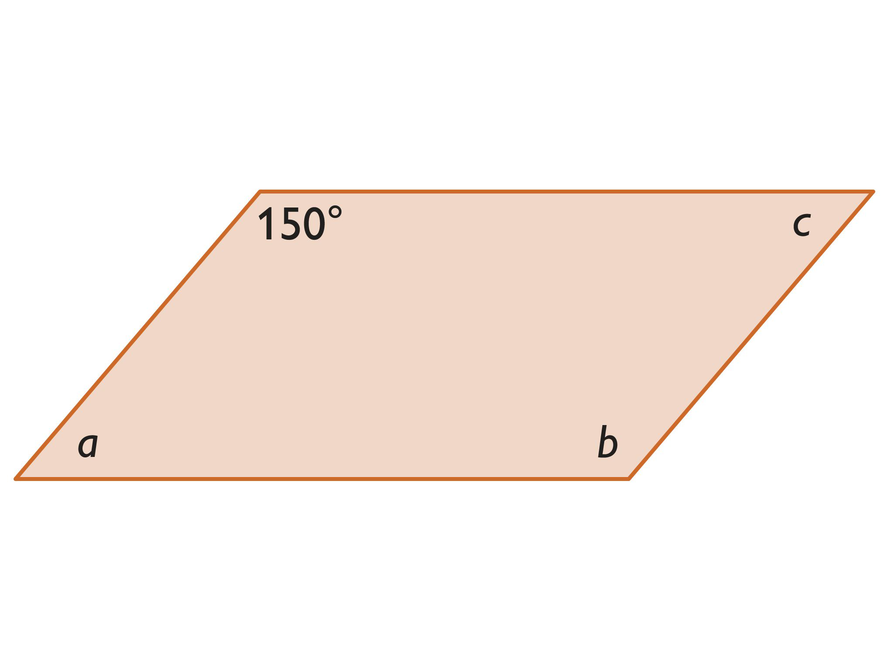## Hint:

• What is the sum of the angles in a parallelogram?
• What do you know about opposite angles in a parallelogram?

# Mathematical Practices

Mathematical Practice 3: Construct viable arguments and critique the reasoning of others.

• Notice students who justify their thinking. During the activity, students will be constructing viable arguments to explain their reasoning.

# Interventions

Student has difficulty getting started.

• What is the angle sum in a triangle?
• What is true of vertical angles and supplementary angles?

Student has a solution.

• Can you explain how you solved the problem?
• Is there another way to solve it?

• The measure of angle a is 65°. The measure of angle b is 50°. The measure of angle c is 130°. The measure of angle d is 50°.
• Angle c is vertical and congruent to the 130° angle. Angles b and d are supplementary to the 130° angle. Angle a is 65° and can be found by adding angle b and the 65° angle, then subtracting the total from 180° (angle sum of a triangle).

# Angles of a Triangle

• What are the measures of ∠a, ∠b, ∠c, and ∠d?
• Explain how you determined each angle measure.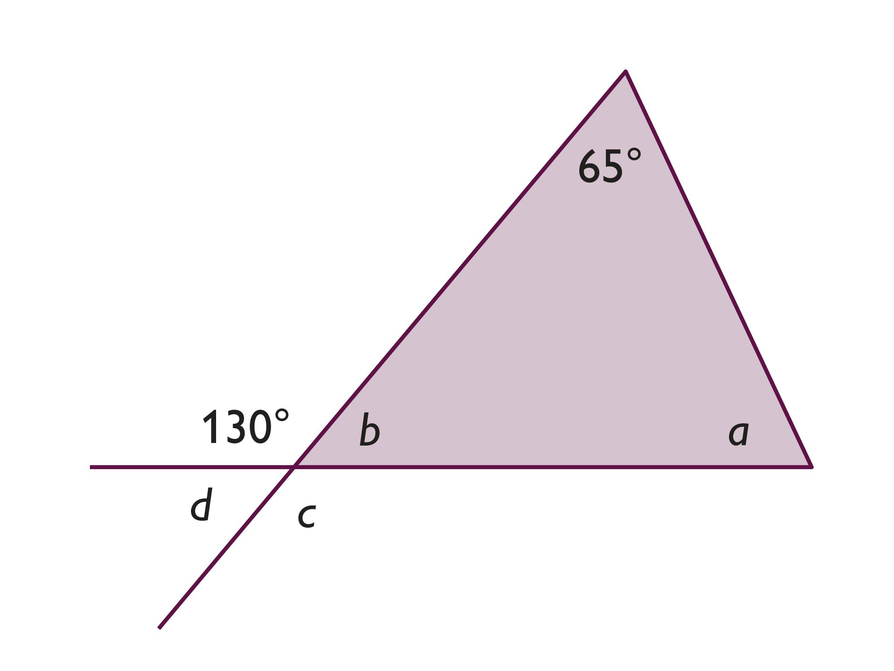## Hint:

• What do you know about vertical angles?
• What do you know about supplementary angles?
• What do you know about the sum of the angles in a triangle?

# Mathematical Practices

Mathematical Practice 8: Look for and express regularity in repeated reasoning.

• Students apply the knowledge they have gained about types of angles and measures of the angles of polygons.

# Interventions

Student has difficulty getting started.

• What is the angle sum in a triangle?

Student cannot solve for x.

• What is the sum of the x-variables? How many x-variables are there?
• What total number of degrees do those x-variables represent?

• x = 22.5°
• In a right triangle, the two non-right angles are complementary.
3x + x = 90°
4x = 90°x = 22.5°

# Angles of a Right Triangle

• Solve for x.
• Explain how you solved for x.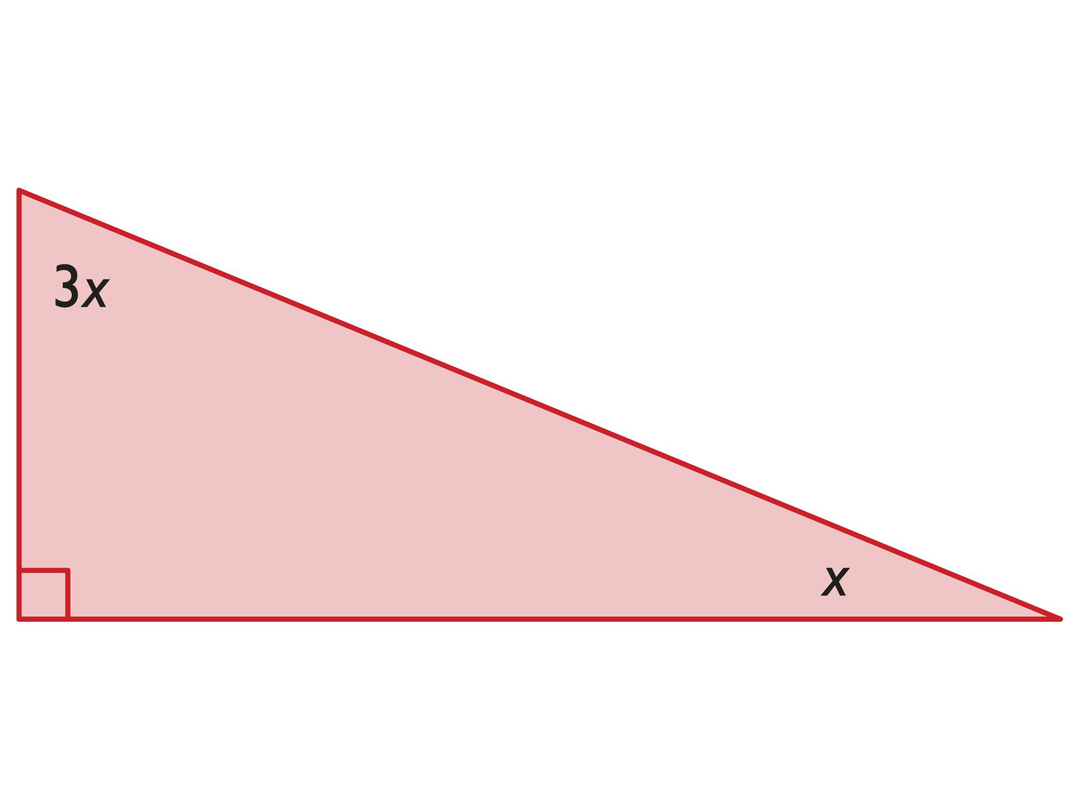## Hint:

• What do you know about the sum of the angles in a triangle?
• What do you know about ∠ a ?

# Interventions

Student has difficulty getting started.

• What do you know about the angles in a parallelogram?
• What is the angle sum in a quadrilateral?

Student has a solution.

• Can you explain how you solved the problem?
• Is there another way to solve it?

• x = 20°
• Solutions will vary. Possible solutions: A rhombus is a parallelogram, so opposite angles are congruent: 7x = 140°x = 20°. Since a rhombus has congruent sides, an isosceles triangle is formed with congruent base angles.

x + 7x + x = 180°

9x = 180°

x = 20°

# Find Missing Angle Measures

• Solve for x.
• Explain how you solved for x.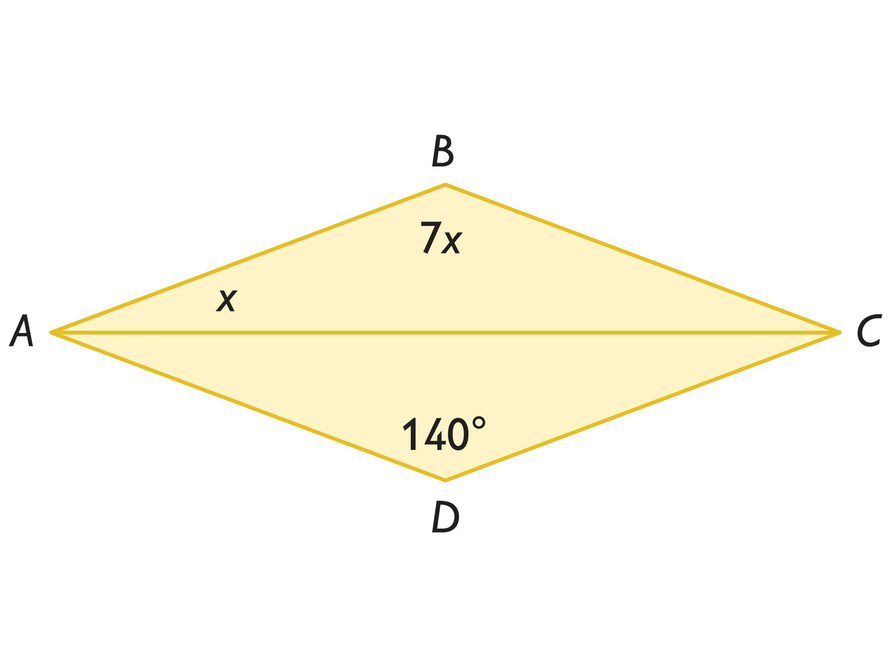## Hint:

• What do you know about the angles in a rhombus?
• What kind of triangle is △ ABC ? What do you know about the angles in this type of triangle?

# Interventions

Student has difficulty getting started.

• What is the angle sum in a pentagon?

Student cannot solve for x.

• What is the sum of the x-variables; how many x-variables are there?
• What total number of degrees do those x-variables represent?

• x = 120°
• A pentagon has an angle sum of 540°. The sum of the measures of two right angles is 180° (90° + 90° = 180°). Since a pentagon has an angle sum of 540°, the sum of the measures of the other three angles must be 360° (540° − 180° = 360°).

x + x + x = 360°

(teacher preference) 3x = 360°

x = 120°

# Find Angles in a Pentagon

• Solve for x.
• Explain how you solved for x.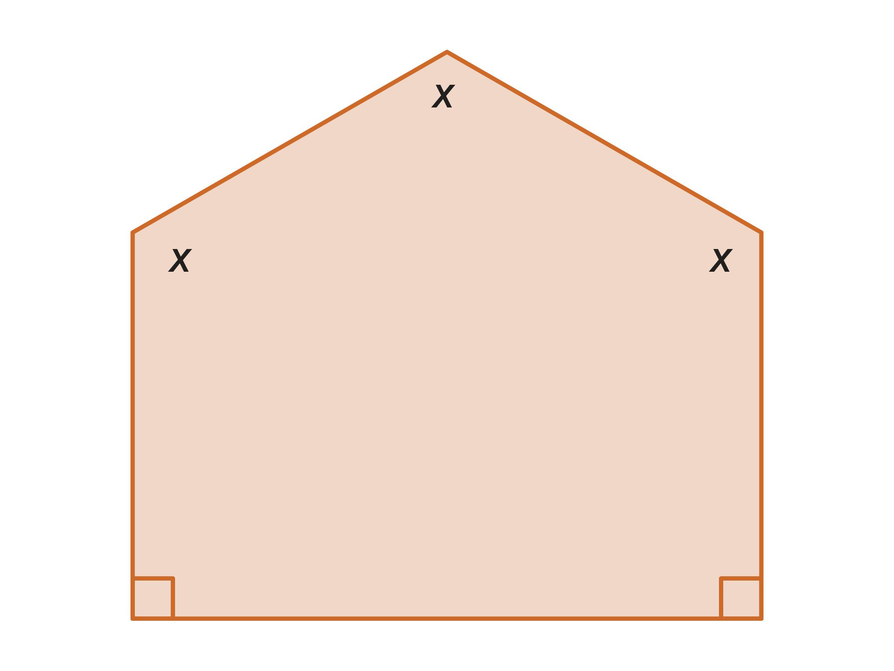## Hint:

• What do you know about the angles in a pentagon?
• If all of the angles are x degrees, what do you know about the angles?

# Interventions

Student has difficulty getting started.

• What is the angle sum in a triangle? A pentagon?
• How can you find the measure of an angle in a regular pentagon?

• The measure of angle a is 36°. The measure of angle b is 72°. The measure of angle c is 72°.
• Since this is a regular pentagon, the angle sum is 540°, and each angle of the pentagon measures 108°. Each of the side triangles are isosceles because two sides are congruent, so the base angles are congruent and each measures 36° (180° − 108° = 72°, 72° ÷ 2 = 36°). The sum of the measures of angle b and one of the 36° angles is 108° (108° − 36° = 72°) and angle b measures 72°. Similarly, angle c measures 72°. This leaves 36° for angle a since 72° + 72° + 36° = 180°.

# Find Angles in the Figure

This figure is a regular pentagon.

• What are the measures of ∠a, ∠b, and ∠c?
• Explain how you determined each angle measure.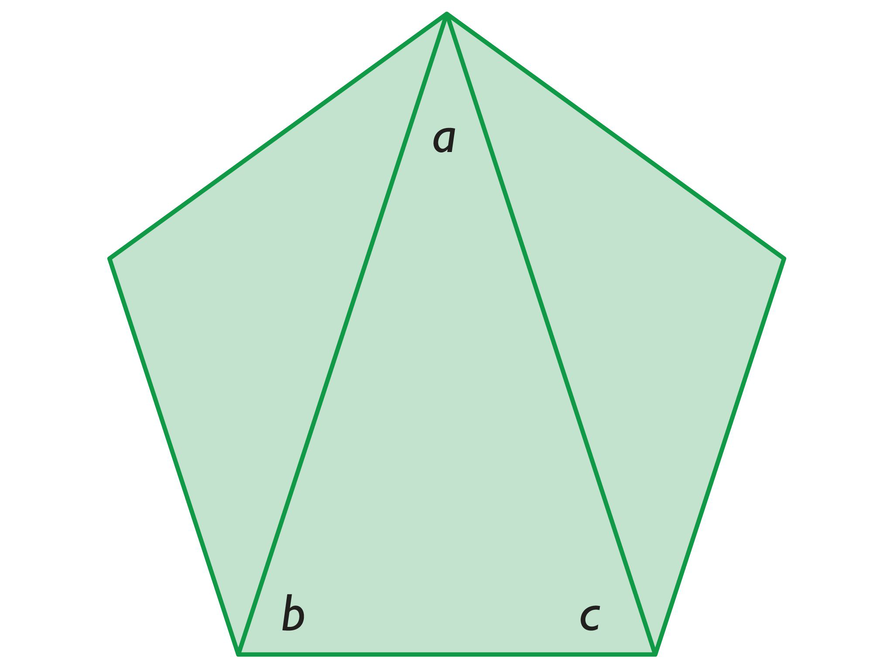## Hint:

What do you know about the angles in a regular pentagon?

# Preparing for Ways of Thinking

Look for these types of responses to share during the Ways of Thinking discussion. Look for students who:

• Use different methods to solve for the angle measures.
• Understand the Challenge Problem and can explain how they solved it, perhaps by drawing the diagonals first.

# Challenge Problem

• The figure is a kite, which will be explored further in the Gallery.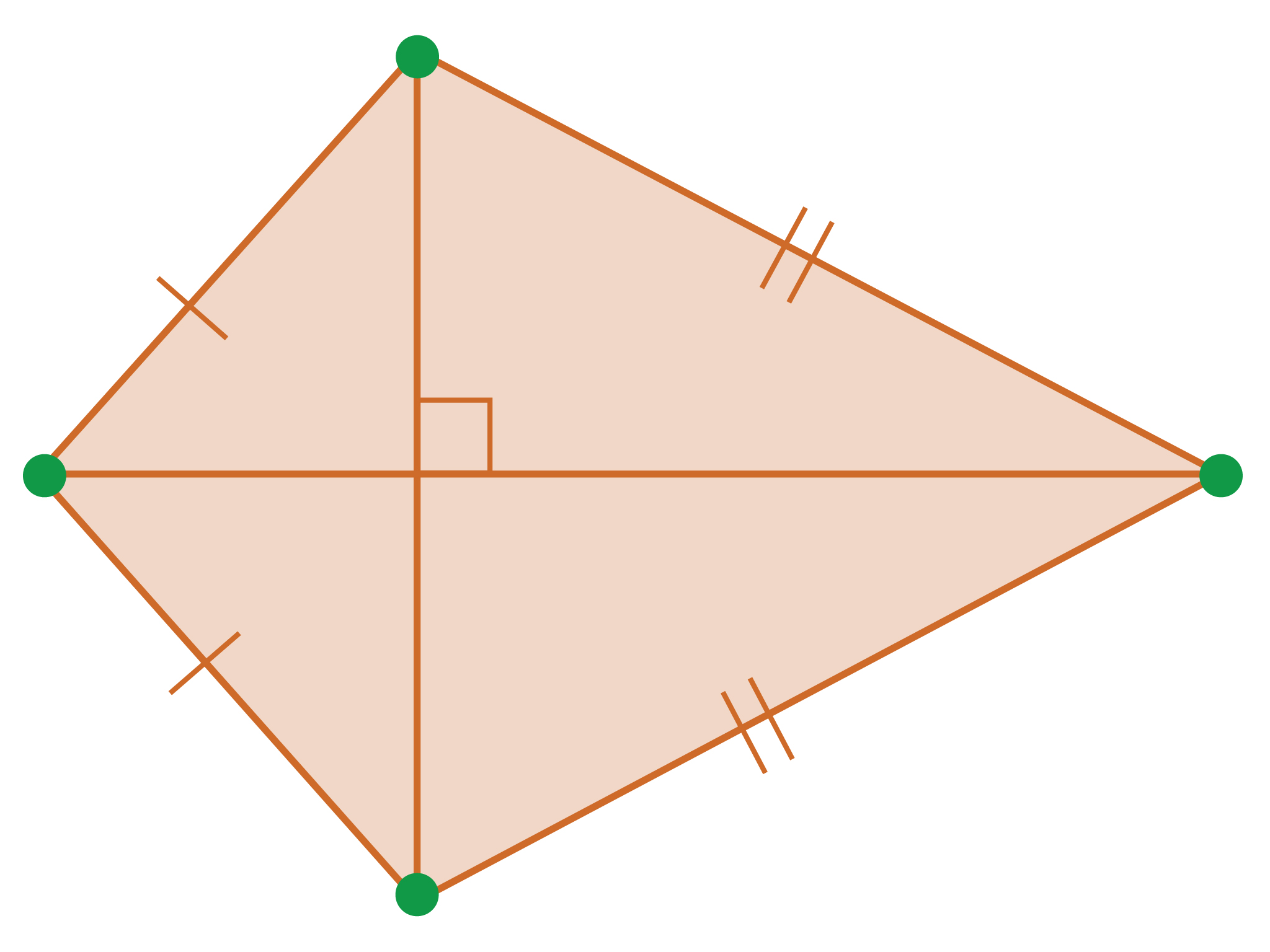# Prepare a Presentation

• Select two of the previous problems: the one you had the most difficulty with, and the one that was easiest for you. Prepare a presentation about your solutions.
• Be prepared to explain and justify each step of your solutions.

# Challenge Problem

• Imagine a quadrilateral that has two pairs of congruent sides with diagonals that are perpendicular to each other. However, the quadrilateral is not a rhombus. Can you draw this figure?

# Mathematics

Facilitate the discussion to help students understand the mathematics of the lesson. Ask questions such as the following:

• What “tools” did you use to solve each of problems?
• How did you decide what to do first in order to solve the problem?
• How did the definitions and characteristics of different figures help you?
• What method can you use to find the angle sum of any polygon?
• What do you know about the angles in a parallelogram?
• What is true about vertical angles and supplementary angles?
• How was the mathematics represented differently by [students]? What was the same? What was different?
• How did [students] make sense of the problem?
• Can you state what [students] said in a different way?

ELL: When selecting a group of students to present during the “Ways of Thinking” section, ensure students present a topic they are confident about. Have students draw diagrams or demonstrate their knowledge in some other way than through verbal language alone.

# Ways of Thinking: Make Connections

Take notes about your classmates' strategies and explanations for finding the missing angle measures.

## Hint:

• What do you know about the angles in a parallelogram?
• What is the sum of the angles in a triangle/quadrilateral/pentagon?
• What is true about vertical angles and supplementary angles?
• How did you decide what to do first in order to solve the problem?
• Is there another way to solve the problem?
• What total number of degrees do the x -values represent?

# A Possible Summary

The properties of vertical, supplementary, adjacent, and complementary angles all play a part in describing geometric figures. Some of the characteristics that describe triangles and quadrilaterals, including how their angles are related to each other, are based on these types of angles. Using this information, you can find the measures of missing angles.

# Summary of the Math: Angles in Polygons

Write a summary about finding missing angle measures in polygons.

## Hint:

• Do you explain how you can use the relationships of angles in different types of polygons to find missing angles?
• Do you describe some things you know about the angles in different types of polygons?

# Lesson Guide

This task allows you to assess students’ work and determine what difficulties they are having. The results of the Self Check will help you determine which students should work on the Gallery and which students would benefit from review before the assessment. Have students work on the Self Check individually.

# Assessment

Have students submit their work to you. Make notes on what their work reveals about their current levels of understanding and their different problem-solving approaches.

Do not score students’ work. Share with each student the most appropriate Interventions to guide their thought process. Also note students with a particular issue so that you can work with them in the Putting It Together lesson that follows.

# Interventions

Check questions that are related to this work.

Student does not calculate m∠1 correctly.

• What is the sum of the angle measures in a triangle?
• What is the sum of the angle measures that you know?

Student does not calculate m∠2 correctly.

• How can you find the measure of ∠FGE?
• What is the sum of the angle measures in a triangle?

Student does not see that ∠3 and ∠BGC (27°) are supplementary angles.

• What type of angle do ∠BGC and ∠CGE form?

Student provides a poor explanation, such as explaining calculations rather than giving mathematical reasons.

• What is the sum of the angle measures in a triangle?
• What do you know about the angles formed by two intersecting lines?

Student provides adequate solution to all questions

• Find a different way of solving the problem.

1. The measure of ∠1 is 63°.
The measure of ∠2 is 135°.
The measure of ∠3 is 153°.
The measure of ∠4 is 45°.
The measure of ∠5 is 27°.

2. Solutions will vary. Students may use the following concepts to find the angle measures:

• Complementary angles to find m∠1
27° + m∠1 = 90°
m∠1 = 63°

• The angle sum of a triangle is 180° to find m∠2 (and m∠1).
18° + 27° + m∠2 = 180°
m∠2 = 135°

•  Supplementary angles to find m∠3
27° + m∠3 = 180°
m∠3 = 153°

• Consecutive angles of a parallelogram are supplementary to find m∠4.
m∠2 + m∠4 = 180°
135° + m∠4 = 180°
m∠4 = 45°

• Opposite angles of a parallelogram are congruent to find m∠5.
18° + m∠5 = m∠4
18° + m∠5 = 45°
m∠5 = 27°

3. The sum of the angles in the hexagon is 720°. There are 6 sides, so use the general rule:

s = (6 − 2)180°

s = 4⋅180°

s= 720°

Students could also add together the measures of each of the angles of the hexagon angles to find the sum.

# Self Check: Analyzing a Hexagon

Complete this Self Check by yourself.

The large figure is a hexagon. Figures ABGF andCDEF are parallelograms.

1. Find the measures of ∠1, ∠2, ∠3, ∠4, and ∠5.
2. Explain how you determined each angle measure.
3. What is the sum of the angles in the hexagon? How do you know?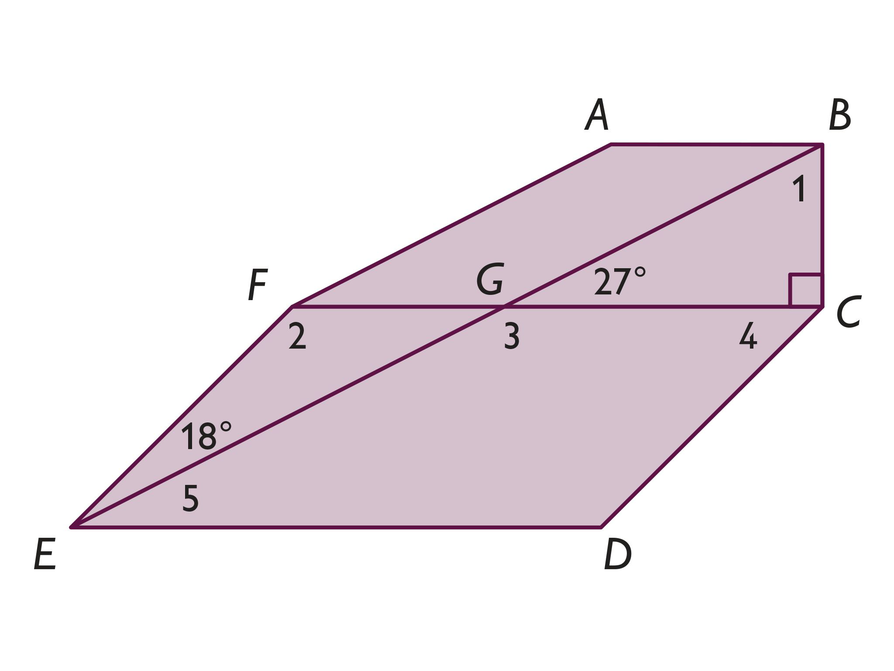# Lesson Guide

Have each student write a brief reflection before the end of class. Review the reflections to get an idea of the connections that students see between triangles, quadrilaterals, and other polygons.

ELL: The “Reflect On Your Work” section provides opportunities for ELLs to develop literacy in English and proficiency in mathematics. Make sure students use both academic and specialized mathematical language when reflecting on their learning at the end of each session. Give students time to discuss the summary before they write.

# Reflection

Write a reflection about the ideas discussed in class today. Use the sentence starter below if you find it to be helpful.

When I look at triangles, quadrilaterals, and other polygons, I see these connections …